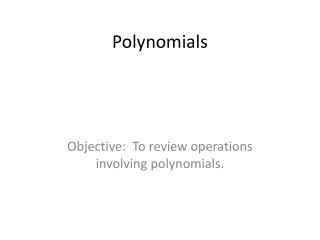# Polynomials - PowerPoint PPT PresentationDownload PresentationPolynomials

PolynomialsDownload Presentation## Polynomials

- - - - - - - - - - - - - - - - - - - - - - - - - - - E N D - - - - - - - - - - - - - - - - - - - - - - - - - - -
##### Presentation Transcript

1. Polynomials Objective: To review operations involving polynomials.

2. Definition • Let be real numbers and let n be a nonnegative integer. A polynomial in x is an expression of the form where . The polynomial is of degree n, is the leading coefficient, and is the constant term.

3. Polynomials • A polynomial with one term is called a monomial. • A polynomial with two terms is called a binomial. • A polynomial with three terms is called a trinomial. • In standard form, a polynomial is written with descending powers of x.

4. Example 1 PolynomialStandard formDegree a) 7 b) 2 c) 8 8 0

5. Operations with Polynomials • You can add and subtract polynomials in much the same way you add and subtract real numbers. Simply add or subtract the like terms (terms having the same variables and the same powers) by adding or subtracting their coefficients.

6. Example 2 • Add or subtract the following polynomials. • a)

7. Example 2 • Add or subtract the following polynomials. • a) • Put the like terms together and add their coefficients.

8. Example 2 • Add or subtract the following polynomials. • You Try • b)

9. Example 2 • Add or subtract the following polynomials. • You Try • b) • Put the like terms together and add their coefficients.

10. Products • To find the product of two polynomials, you can use the distributive method. If you are multiplying two binomials, you can also FOIL to find the answer.

11. Example 3 • Multiply the following binomials.

12. Example 3 • Multiply the following binomials. • First, the distributive method.

13. Example 3 • Multiply the following binomials. • First, the distributive method. • Now, FOIL

14. Example 3 • Multiply the following binomials. • You Try.

15. Example 3 • Multiply the following binomials. • You Try.

16. Example 4 • When multiplying two trinomials, you must use the distributive method and collect like terms. It is easiest to do this using a vertical arrangement.

17. Example 4 • When multiplying two trinomials, you must use the distributive method and collect like terms. It is easiest to do this using a vertical arrangement. • Multiply

18. Example 4 • When multiplying two trinomials, you must use the distributive method and collect like terms. It is easiest to do this using a vertical arrangement. • Multiply

19. Example 4 • When multiplying two trinomials, you must use the distributive method and collect like terms. It is easiest to do this using a vertical arrangement. • Multiply

20. Example 4 • Multiplying two trinomials. • You Try.

21. Example 4 • Multiplying two trinomials. • You Try.

22. Example 5 • Multiplying the sum and difference of the same terms.

23. Example 5 • Multiplying the sum and difference of the same terms.

24. Example 5 • Multiplying the sum and difference of the same terms.

25. Example 5 • Multiplying the sum and difference of the same terms.

26. Example 5 • Multiplying the sum and difference of the same terms. • You Try:

27. Example 5 • Multiplying the sum and difference of the same terms. • You Try:

28. Example 6 • Squaring a Binomial.

29. Example 6 • Squaring a Binomial.

30. Example 6 • Squaring a Binomial.

31. Example 6 • Squaring a Binomial.

32. Example 6 • Squaring a Binomial. • You Try:

33. Example 6 • Squaring a Binomial. • You Try:

34. Example 7 • Cube of a Binomial.

35. Example 7 • Cube of a Binomial.

36. Example 7 • Cube of a Binomial.

37. Example 7 • Cube of a Binomial. • You Try:

38. Example 7 • Cube of a Binomial. • You Try:

39. Homework • Pages 29-30 • 3-21 multiples of 3 • 30-45 multiples of 3 • 48-69 multiples of 3